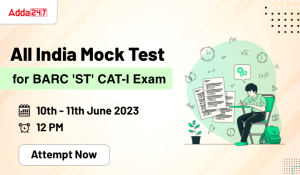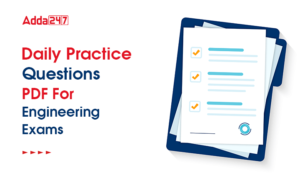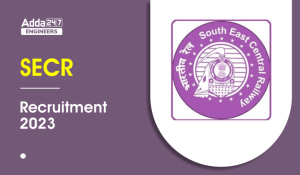Engineering Jobs   »   Civil Engineering quizs   »   UPSSSC JE QUIZ

# UPSSSC-JE’21 CE: Daily Practice Quiz. 30-September-2021

Know your strengths and practice your concepts with this quiz on UPSSSC-JE Recruitment 2021. This quiz for UPSSSC-JE Recruitment 2021 is designed specially according to UPSSSC-JE Syllabus 2021.

Quiz: Civil Engineering
Exam: UPSSSC-JE
Topic: MISCELLANEOUS

Each question carries 1 mark
Negative marking: 1/4 mark
Time: 8 Minutes

Q1. Viscosity of a fluid with specific gravity 1.3 is measured to be 0.0034 Ns/m². Its kinematic viscosity, in m²/s, is___________:
(a) 2.6 × 10-6
(b) 4.4 × 10-6
(c) 5.8 × 10-6
(d) 7.2 × 10-6

Q2. Tesla is the SI unit of _________
(a) magnetic flux density
(b) magnetic flux
(c) luminous flux
(d) illumination

Q3. Two Tensile forces, each of magnitude F are acting at a point perpendicular to each other, then their resultant force will be :
(a) √F
(b) √2F
(c) √2 F
(d) Zero

Q4. What are the equilibrium conditions to be satisfied for a particle applied with a system of non-coplanar, concurrent forces?
(a) ΣF_x = 0 & ΣF_y = 0
(b) ΣF_x = 0, ΣF_y = 0] ΣF_z = 0, ΣM_x = 0m ΣM_y = 0 & ΣM_z = 0
(c) ΣF_x = 0, ΣF_y = 0 & ΣF_(z-axis) = 0
(d) ΣF_x = 0, ΣF_y = 0 & ΣF_z = 0

Q5. Angle of friction is the
(a) Angle between normal reaction and the resultant of normal reaction and the limiting frictional force
(b) Ratio of limiting friction and normal reaction
(c) Ratio of static and dynamic friction
(d) None of the above

Q6. A flat road has a curve segment with a radius of 150 m. While negotiating this curve, vehicle slipped on its tyres as well as tried to roll over at a particular speed. This speed assuming a friction coefficient of 0.4 is :
(a) 18 m/s
(b) 25 m/s
(c) 32 m/s
(d) 20 m/s

SOLUTION

S1. Ans. (a)
Sol. Dynamic viscosity (μ) = 0.0034 (N-S)/m²
Specific gravity (G) = 1.3
Kinematic viscosity (ν) = μ/ρ
ν = μ/(ρ_w G)
ν = 0.0034/(1000×1.3 )
= 2.61 × 10^(-6) m²/sec.

S2. Ans.(a)
Sol. Tesla is the unit of magnetic flux density in SI.

S3. Ans.(c)
Sol. R = √(F^2+F^2+2F.Fcos 90°)
R = √2 F

S4. Ans.(d)
Sol. the sum of all forces in three direction should be zero the moment should also be zero but in case of concurrent forces moment will be automatically zero.

S5. Ans.(a)Sol. The angle of friction is the angle between the resultant of friction force and normal reaction force with Normal reaction force.
In this case frictional force have its maximum value i.e limiting friction force.

S6. Ans.(b)
Sol. In case of road turn the centrifugal force should be equal to friction force

Sharing is caring!

•GATE Result 2023 Out, Download Result, C...
•GATE 2023 Admit Card Out, Download Link ...
•All India Mock Test for BARC 'ST' CAT-I ...
•DDA JE Recruitment 2023 Out, Apply Onlin...
•BEL Recruitment 2023, Notification, Appl...
•Daily Practice Questions PDF For Enginee...
•SSC JE Result 2023 Out, Direct Download ...
•SECR Recruitment 2023 Out, Apply Online ...